## PromQL之选择器和运算符

news/2023/6/6 23:23:05
1. 平台统一监控的介绍和调研
2. 直观感受PromQL及其数据类型
3. PromQL之选择器和运算符

# PromQL 匹配器

1. 相等匹配器（=）

``````jvm_memory_used_bytes{id="G1 Eden Space"}
``````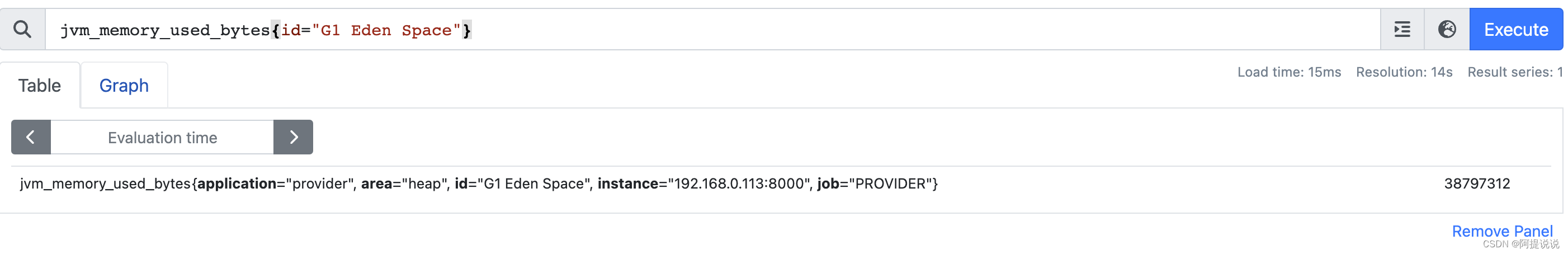1. 不相等匹配器（!=）

``````jvm_memory_used_bytes{id!="G1 Eden Space"}
``````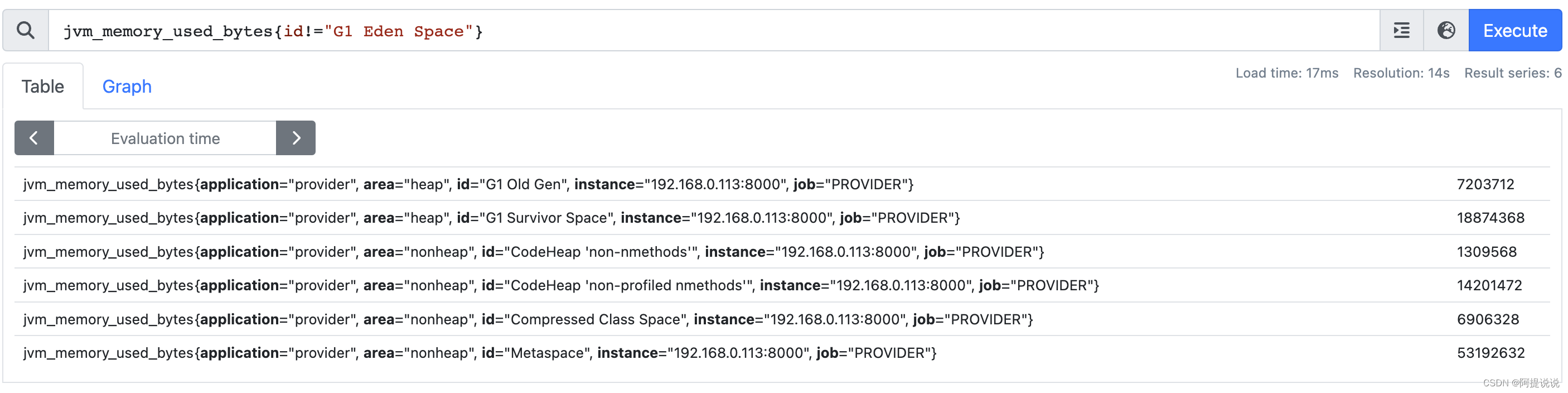1. 正则表达式匹配器(=~)

``````jvm_memory_used_bytes{id =~ "G1.*"}
``````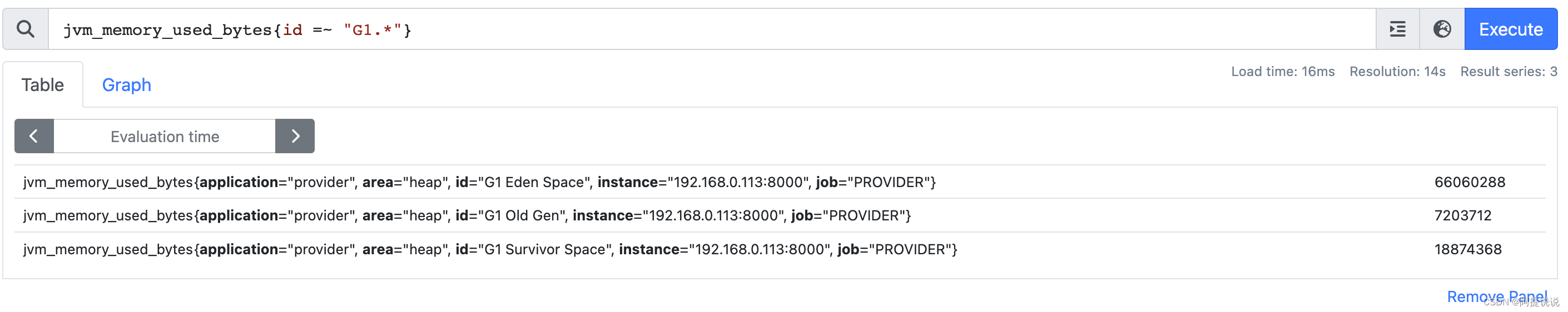1. 不等于的正则表达式匹配器(!~)

``````jvm_memory_used_bytes{id !~ "G1.*"}
``````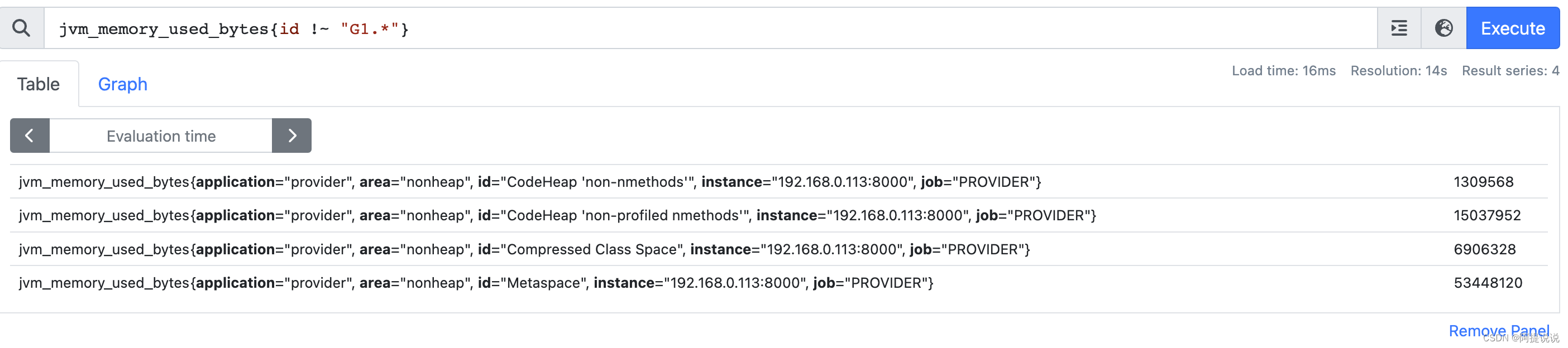jvm_memory_used_bytes 同 {name = “jvm_memory_used_bytes”}，也可以用其他匹配器

# PromQL 选择器

## 瞬时向量选择器

``````jvm_memory_used_bytes
``````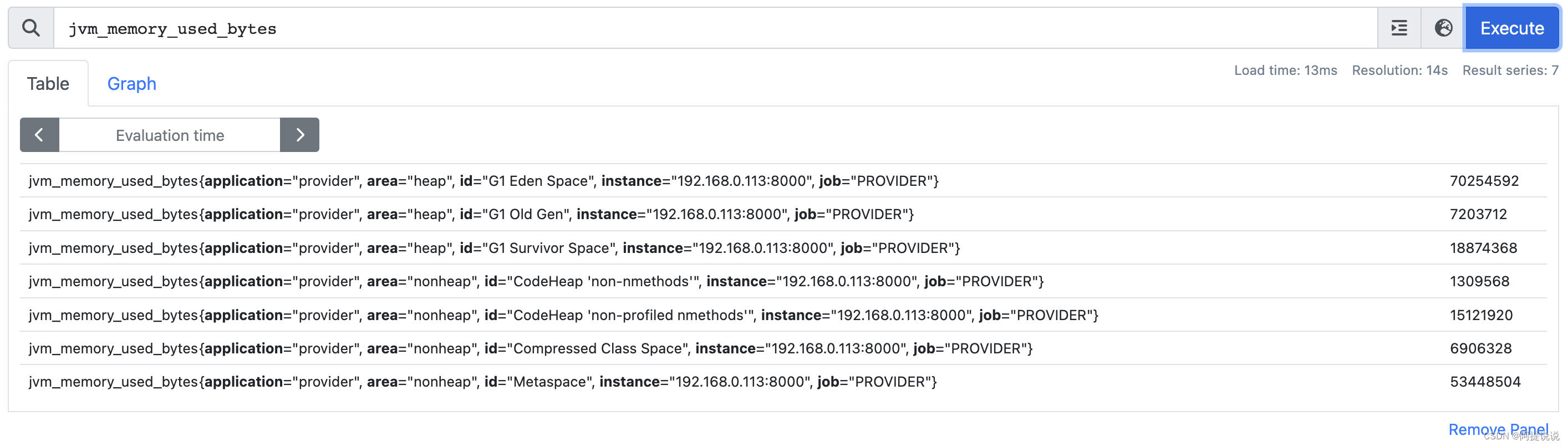## 区间向量选择器

``````jvm_memory_used_bytes[1m]
``````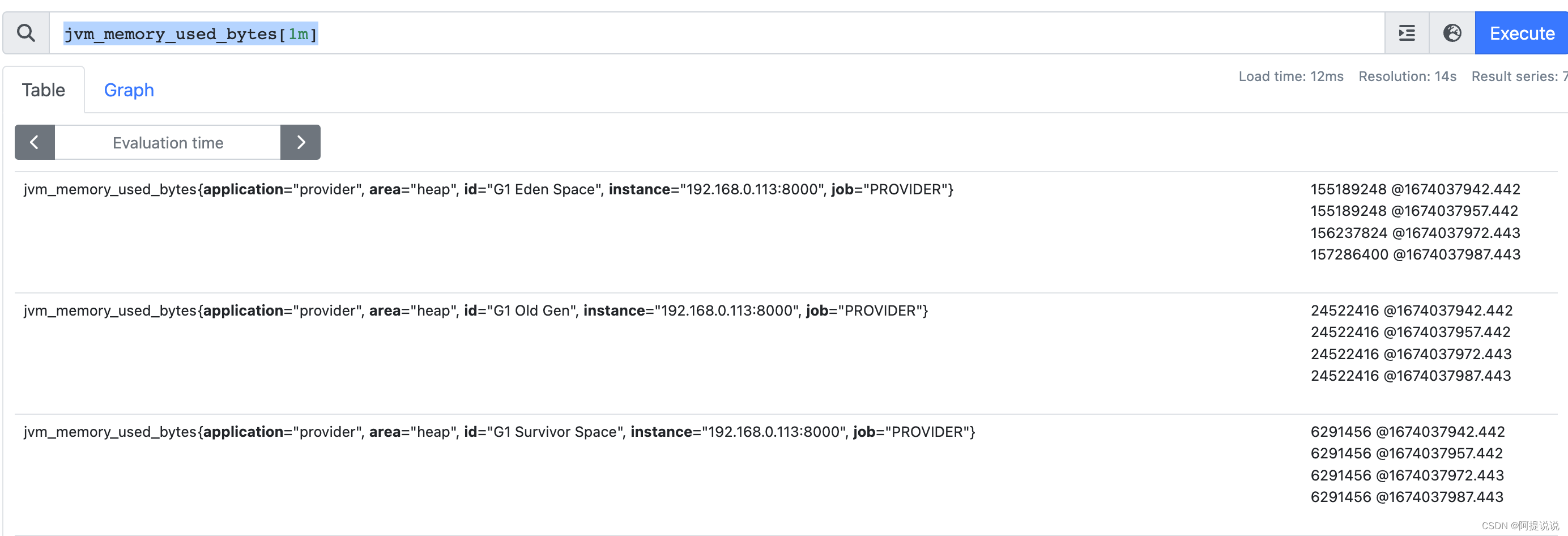## 偏移量修改器

``````jvm_memory_used_bytes offset 1m
``````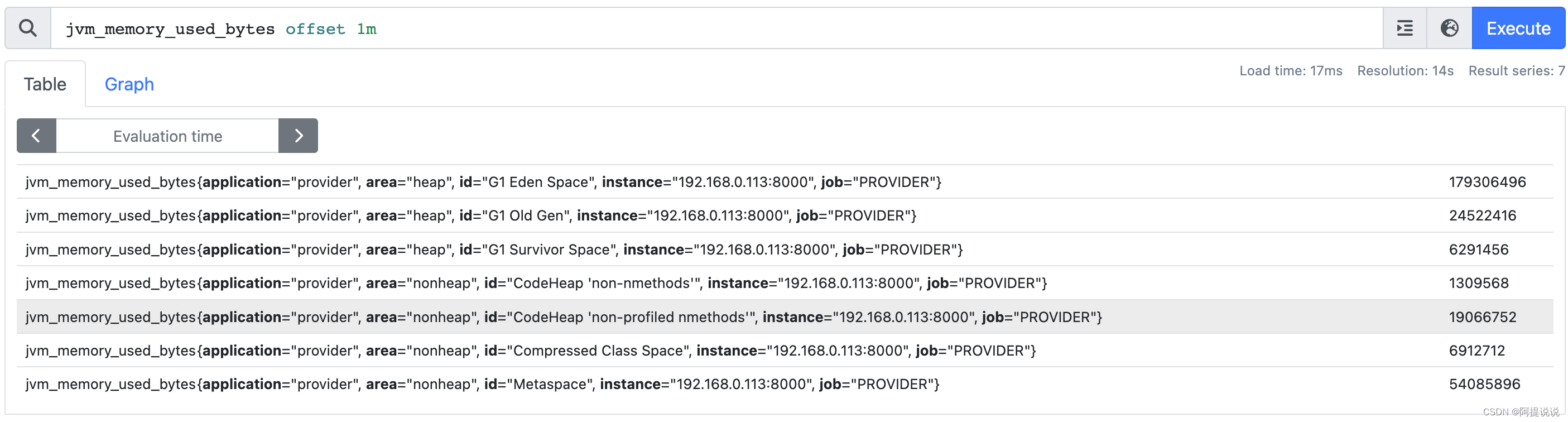## @ 修饰符

@ 修饰符能够修改瞬时向量和区间向量的求值时间，使用@时间戳 表示

``````jvm_memory_used_bytes @1674040139
``````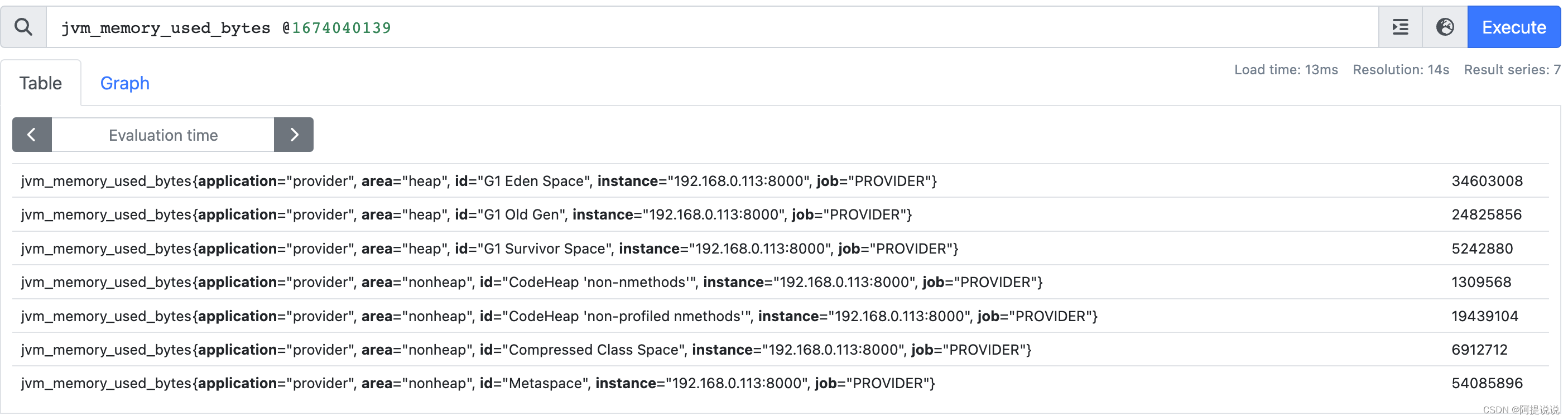``````jvm_memory_used_bytes @1674040139 offset 5m
``````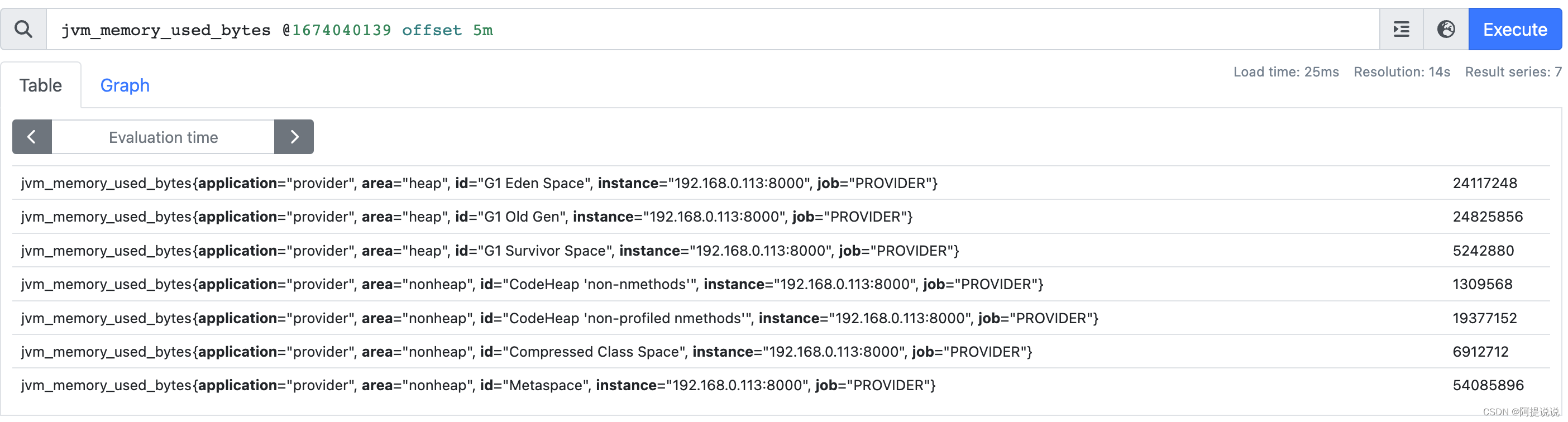# PromQL 运算符

## 算术运算符

sum(jvm_memory_used_bytes{area=“heap”}) 表示已使用的堆内存
sum(jvm_memory_max_bytes{area=“heap”}) 表示堆总内存

``````sum(jvm_memory_used_bytes{area="heap"})*100 / sum(jvm_memory_max_bytes{area="heap"})
``````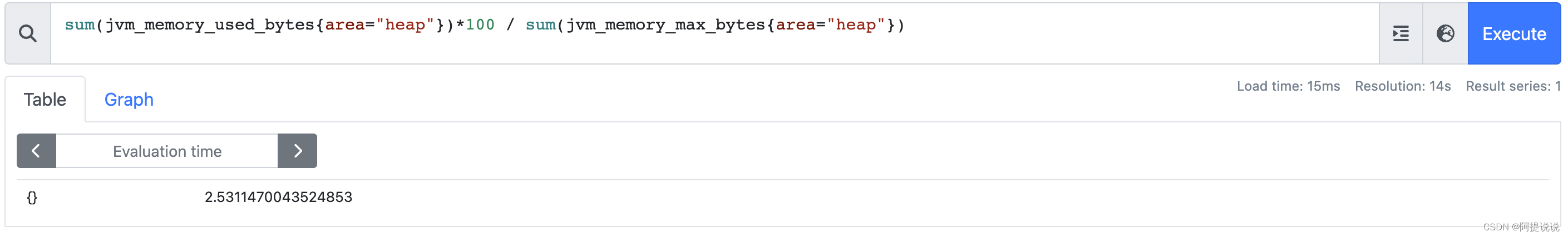## 逻辑运算符

and（并且）、or（或者）、unless（排除）

• vector1 and vector2 ：产生一个新的向量，向量中的元素由vector1完全匹配 vector2的元素组成
• vector1 or vector2：产生一个新的向量，由vector1中的元素 和 vector2中不与vector1匹配的元素 组成
• vector1 unless vector2：产生一个新的向量，由vector1 中没有与vector2匹配的元素组成

and 示例：

vector1：所有实例的堆内存数据

``````jvm_memory_used_bytes{area="heap"}
``````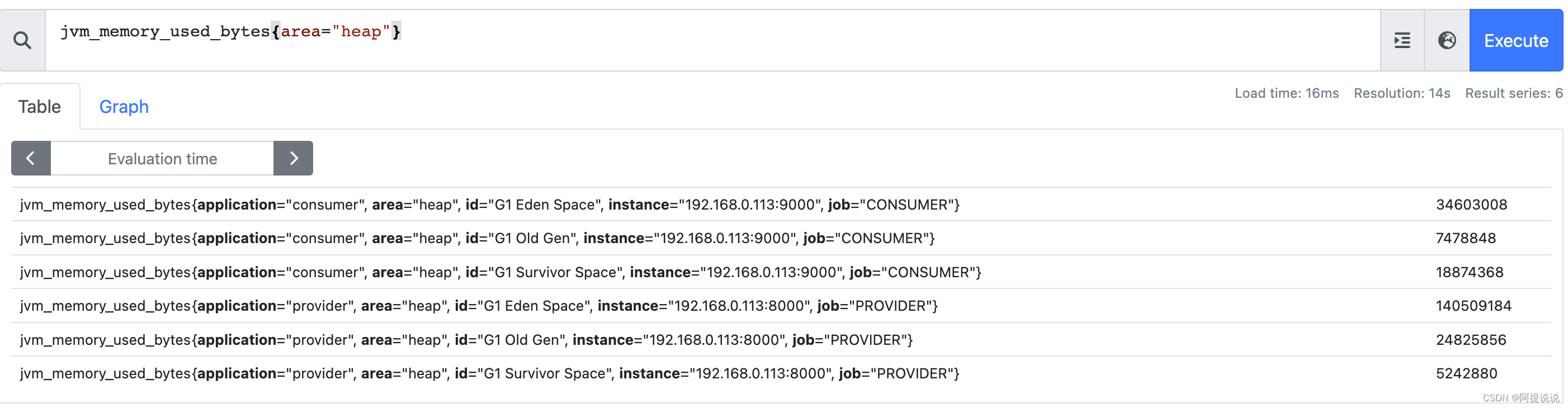vector2：筛选出 instance=“192.168.0.113:9000”,area=“heap” 数据

``````jvm_memory_max_bytes{instance="192.168.0.113:9000",area="heap"}
``````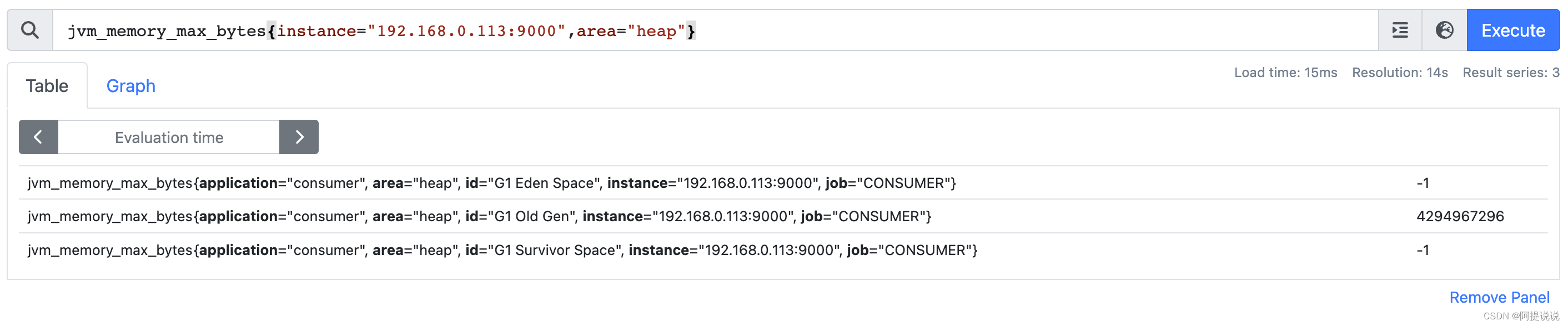and 运算符后，只保留了instance=“192.168.0.113:9000”,area=“heap” 标签相同的数据

``````jvm_memory_used_bytes{area="heap"} and jvm_memory_max_bytes{instance="192.168.0.113:9000",area="heap"}
``````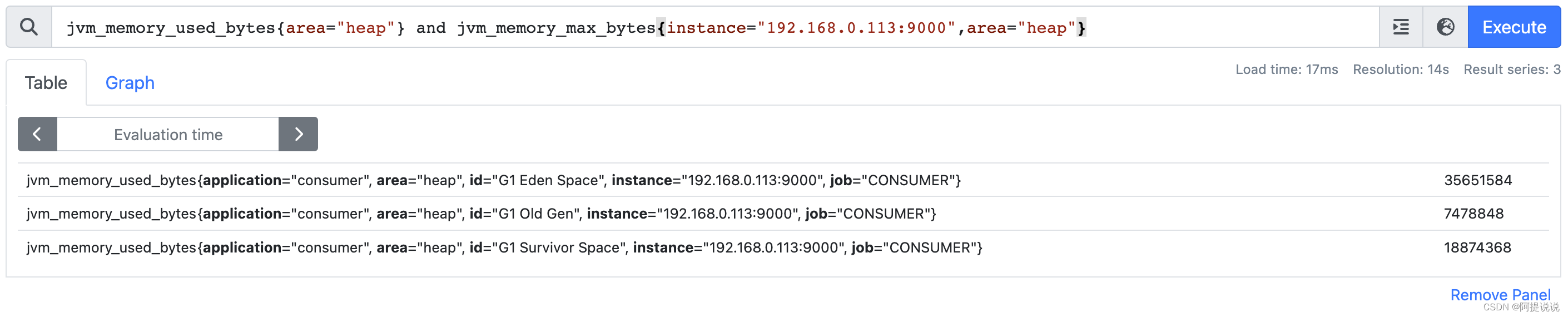or 示例：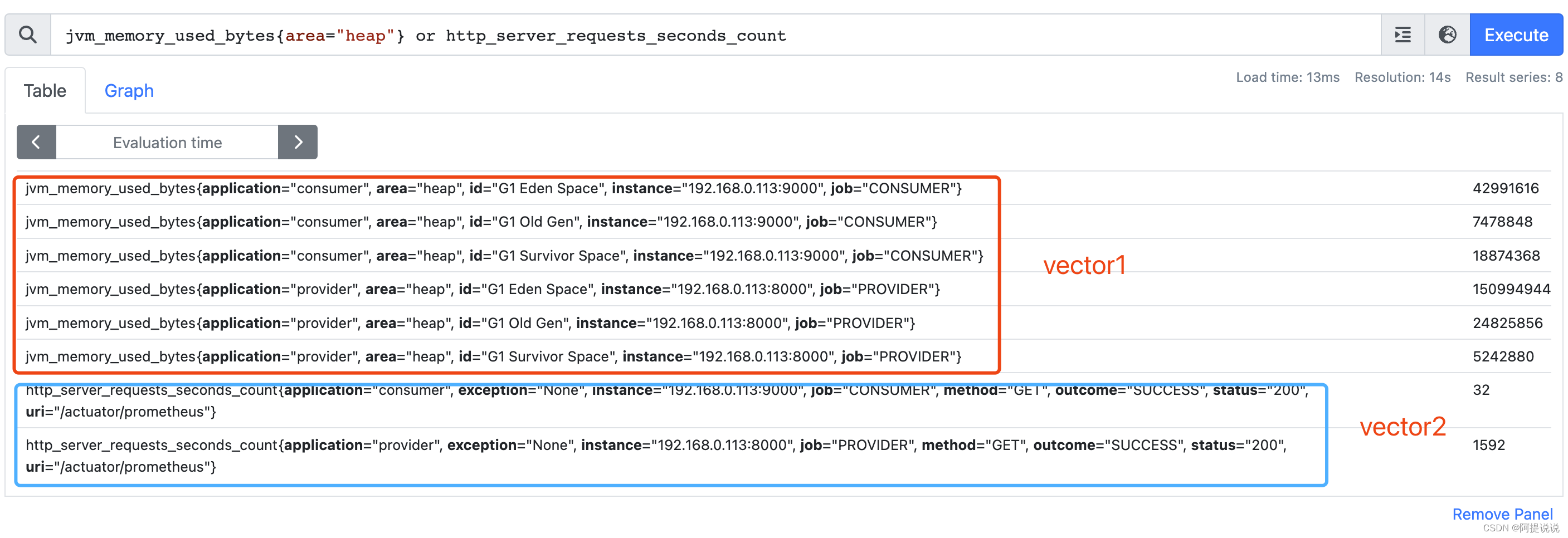unless 示例：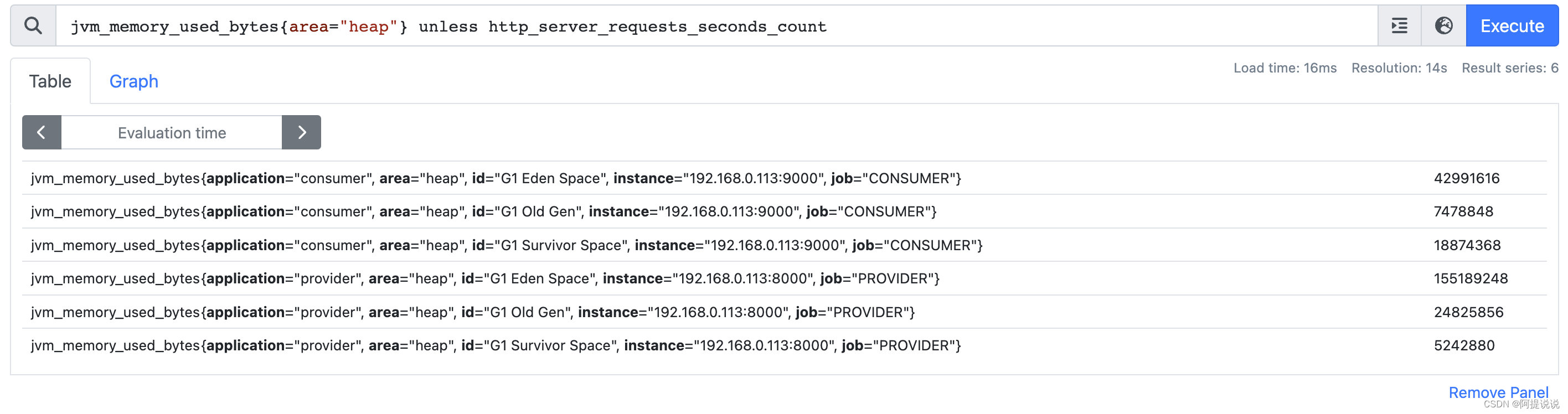## 比较运算符

== 相等、!=不相等、>大于、<小于、>=大于等于、<=小于等于

``````99 >= bool 88
``````

# 向量匹配

Prometheus 的向量与向量 之间进行运算操作时会基于默认的匹配规则：依次找到与左边向量元素匹配（标签完全一致）的右边向量元素进行运算，如果没有找到匹配元素，直接丢弃。向量匹配主要有：一对一，一对多，多对一。

``````process_open_fds / process_max_fds
``````

on：指定要匹配的标签，只匹配指定的标签。

ignoring：忽略某些标签，就是指定的这些标签不匹配，其他的都匹配。

``````sum by(instance, job) (rate(node_cpu_seconds_total{mode="idle"}[5m]))
/ on (instance, job)
sum by(instance, job) (rate(node_cpu_seconds_total [5m] ))
``````

``````process_open_fds < process_max_fds
process_max_fds >  process_open_fds
``````

group_left: 左边有更多的子集
group_right:右边有更多的子集

``````<vector expr> <bin-op> ignoring(<label list>) group_left(<label list>) <vector expr>
<vector expr> <bin-op> ignoring(<label list>) group_right(<label list>) <vector expr>
<vector expr> <bin-op> on(<label list>) group_left(<label list>) <vector expr>
<vector expr> <bin-op> on(<label list>) group_right(<label list>) <vector expr>
``````

Grafana 系列文章，版本：OOS v9.3.1

1. Grafana 的介绍和安装
2. Grafana监控大屏配置参数介绍（一）
3. Grafana监控大屏配置参数介绍（二）
4. Grafana监控大屏可视化图表
5. Grafana 查询数据和转换数据
6. Grafana 告警模块介绍
7. Grafana 告警接入飞书通知

### 使用NLTK对文档进行分句

from nltk.corpus import gutenberg from nltk.tokenize import sent_tokenize text gutenberg.raw("austen-emma.txt") sentences sent_tokenize(text)print(sentences)

### php 文章分句,php 英文分句/分段落

[php]代码库<?php /*TWWYS ART*/function break_passage(\$text){ //分割段落return preg_split("/(\\r|\\n|\\r\\n)/", \$text, -1, PREG_SPLIT_NO_EMPTY);}function break_sentence(\$text){ //分割句子 英文的句号后面必须有空格\$re /# Split sentences on whit…